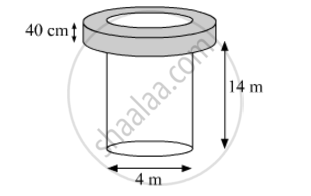# A well of diameter 4 m is dug 14 m deep. The earth taken out is spread evenly all around the well to form a 40 cm high embankment. Find the width of the embankment. - Mathematics

A well of diameter 4 m is dug 14 m deep. The earth taken out is spread evenly all around the well to form a 40 cm high embankment. Find the width of the embankment.

#### SolutionDepth (h1) of the well = 14 m

Radius (r1) of the circular end of the well =4/2 m = 2 m

Height (h2) of embankment = 40 cm = 0.4 m

Let the width of embankment be x.

From the figure, it can be observed that the embankment will be cylindrical in shape having outer radius (r2) as (2 + x) m and inner radius (r1) as 2 m.

Volume of earth dug from the well = Volume of earth used to form embankment

pir_1^2h_1=pi(r_2^2-r_1^2)h_2

=>pi(2)^2 14=pi[(2+x)^2-2^2]0.4

=>4xx14=(x(x+4)4)/10

x2+4x140=0

x2+14x10x140=0

(x+14)(x10)=0

x=10                (Because x cannot be negative)

Therefore, the width of the embankment will be 10 m.

Concept: Concept of Surface Area, Volume, and Capacity
Is there an error in this question or solution?